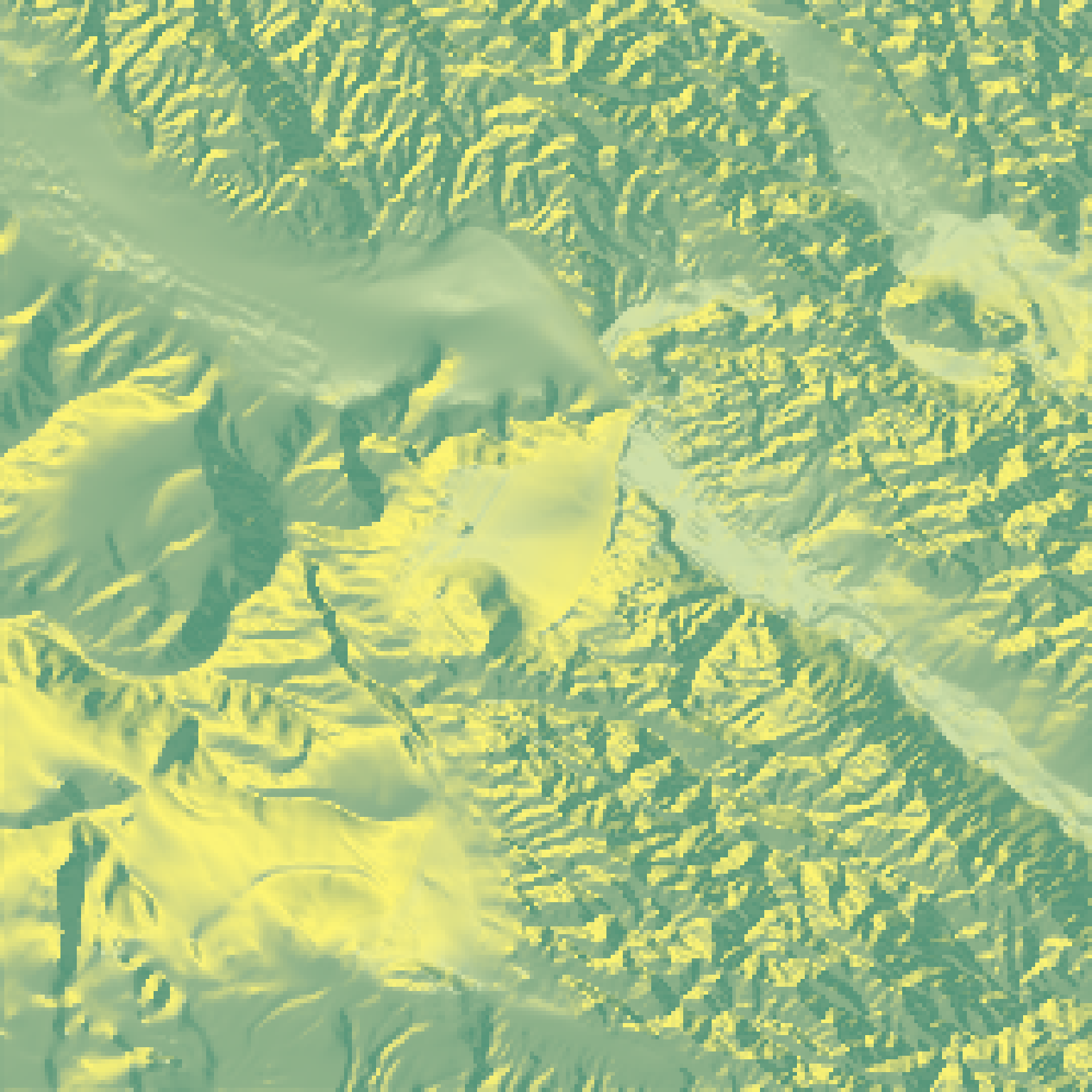Resizes a matrix (preserving contents) by specifying the desired output dimensions or a scaling factor.

resize_matrix(
heightmap,
scale = 1,
width = NULL,
height = NULL,
method = "bilinear"
)

## Arguments

heightmap

The elevation matrix.

scale

Default 0.5. The amount to scale down the matrix. Scales using bilinear interpolation.

width

Default NULL. Alternative to scale argument. The desired output width. If width is less than 1, it will be interpreted as a scaling factor-- e.g. 0.5 would halve the resolution for the width.

height

Default NULL. Alternative to scale argument. The desired output width. If height is less than 1, it will be interpreted as a scaling factor-- e.g. 0.5 would halve the resolution for the height.

method

Default bilinear. Method of interpolation. Alteratively cubic, which is slightly smoother, although current implementation slightly scales the image.

## Examples

#Reduce the size of the monterey bay dataset by half

if(run_documentation()) {
montbaysmall = resize_matrix(montereybay, scale=0.5)
montbaysmall %>%
plot_map()
}if(run_documentation()) {
#Reduce the size of the monterey bay dataset from 540x540 to 100x100
montbaysmall = resize_matrix(montereybay, width = 100, height = 100)
montbaysmall %>%
plot_map()
}if(run_documentation()) {
#Increase the size of the volcano dataset 3x
volcanobig = resize_matrix(volcano, scale=3)
volcanobig %>%